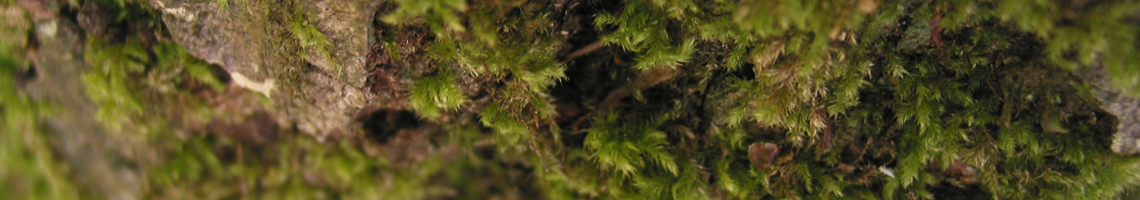# Geometría proyectiva: Involution in overlapping series of second order : Axis of involution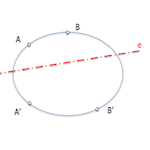The involutionary transformations applications bijective of great interest are to be used in geometric constructions, since they simplify them considerably.

We will see how defined an involution in second-order series, with base a conical, Comparing the new model of projective transformation with the studied in the so-called overlapping series of second order .

We will remember that when determining the is between two superimposed series of second order (basis of a common conical) We started three-point, A, B to C, and their respective counterparts: A’, B’ y C’.

To project the otraserie elements from two homologous points got do perspective whose perspective axis was projective series shaft, called “Straight from Pascal”.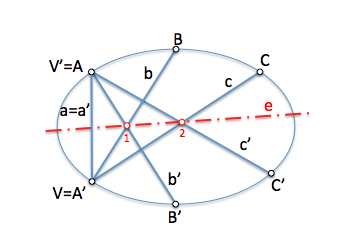To define an involution enseries of second order will have to relate two pairs of points just. In the figure the involution is determined by pairs of homologous elements a-a.’ and b-b’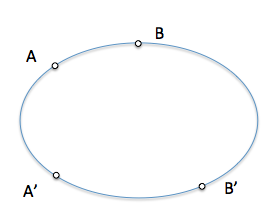This does not mean that we are determining a Conic by four points, but that, given any Cone, If we take four points we can determine an involution of points. Analogous, in the previous case of overlapping series, We were not defining the Conic by six points, We simply residual them proyectivamente.

To tell us that the points a-a.’ and b-b’ they are in involution, they are telling us that there is a dual correspondence between them in a way that, If we consider that about B’ There is another system that we can call “C”, your transformed C’ you will be in the same position as point B.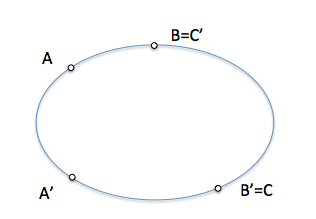We could repeat this idea with A point, Although it is not necessary since we converted the problem of determining the elements of the is in the well-known case, mentioned at the beginning, overlapping series of second order.

We can determine therefore the projective as in the previous case axis, projecting from a point A and its counterpart A’ points B ’-C’ and B-C to determine two bundles perspective. This projective axis is referred to as “Axis of involution

This line will be very useful to operate with the conical.

We can ask ourselves some immediate application problem, as it can be to get a new, either the transformed the fifth point that completes the definition of the Conic.

Get the counterpart of the point “X” in the involution defined by pairs of homologous points a-a. ’, B-B’

The figure has been represented the axis of involution which we previously calculated, eliminating paths to simplify the image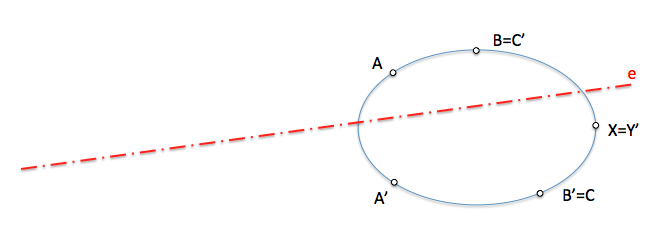We operate as in the case of overlapping series of second order, projecting the point from V ’ = to and finding homologous beam Ray perspective that is trimmed in the projective axis (item (J)) and will need per vertex V = to ’.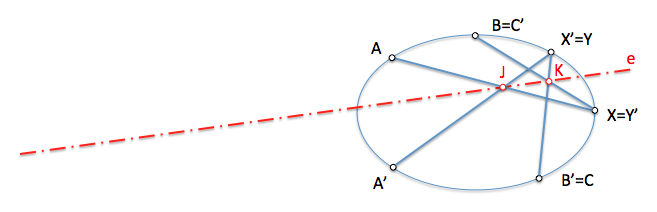The searched point will be therefore the straight a-j. We will have to repeat this procedure, projecting from B and B’ to locate a new straight line in which the searched point is (Intersection of two loci).

Please note that although we have represented the taper to facilitate the interpretation of geometry which we are analysing, This curve is not available in our paths

We have determined the “Axis of involution” and we have used it to determine homologous elements in the projective transformation that defined by. We will see new properties and its use in the determination of the main elements of the Conic, center, diameters, axes, to go forward in the study associated with this interesting transformation.

Projective Geometry